Open in App
Not now

# How to Calculate Percentiles For Monitoring Data-Intensive Systems?

• Difficulty Level : Medium
• Last Updated : 08 Dec, 2021

Monitoring often involves the use of percentiles. Unlike average values, which are heavily influenced by outliers, percentiles help understand how the system works most of the time. If 9 out of 10 requests are executed in 1 second and the last one takes 10 seconds, the average will be 1.9 seconds while the 50th percentile will be 1 second. This is but one example of how the average value is not appropriate for monitoring. Thus, the need to count percentiles arises, and for this very reason, we added a summary collector to our tarantool/metrics. Summary collectors calculate quantiles for the monitored data. Let me tell you about the algorithm we used to compute quantiles and how we implemented it for tarantool/metrics.

### Summary Collector

Algorithm-quantile is a value that a random variable does not exceed with a probability of. For example, in HTTP request monitoring, a 0.5-quantile (basically the 50th percentile) that equals 1-second means that 50% of requests were processed in less than a second. To calculate afor a sorted array of size n, you need to find the element with the index of. This approach requires storing all the monitored data, and there can be a lot of data in metrics. If there are one billion requests to be processed, they would require a billion array elements, which would make up about 1 GB of data.

This problem can be solved by a number of algorithms that calculate approximate quantile values for data streams. We took the algorithm used in Prometheus. It compresses the original data, representing them as a set of segments. Each segment is described by a structure of three numbers:is the distance from the beginning of the previous segment to the beginning of the current segment;is the length of the current segment;is the approximate quantile of the segment.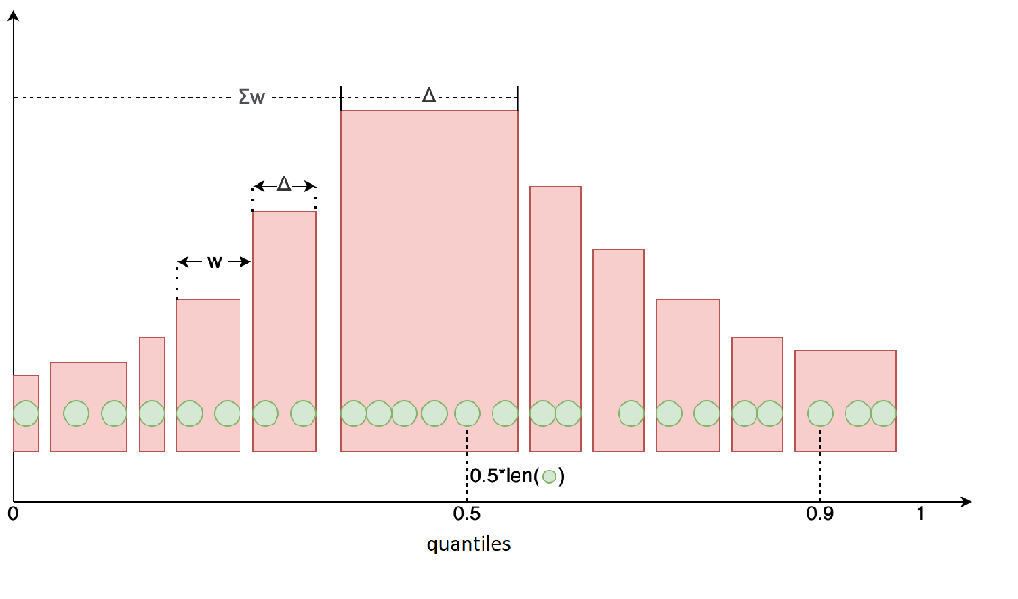The graph above shows the original array elements in green and the compressed array elements in red. To find the quantile for the compressed data, we need to iterate over the segments, adding up their distances until the sum is close enough to, and identify the corresponding segment. For example, the 0.5-quantile will be located in the middle of the green array on the graph, and the approximated value will belong to the corresponding red segment. The whole compression process is described extensively in the original article.

### Implementation

We followed the example of the Go implementation of this algorithm. Let’s create two arrays. One will serve as a buffer for the monitored values, and the other will be used as an observation array to store segment structures:

## Go

 typedef struct {int Delta, Width; double Value; } sample;

This algorithm operates only on sorted values. Let’s limit the buffer size to 500 values and define the size of the observation array as 2 × 500 + 2. As compression reduces the array size by approximately half, we’ll need on average 500 elements of the uncompressed array from the previous step + 500 elements added to the array in the current step + elements liketo simplify searching in the array.

### Development

We worked on our implementation iteratively: created a version, checked its performance with a profiler, compared it with the Go version, then looked for ways to improve it. We assessed our results using a simple benchmark: 108 samples, which takes about 8 seconds for the Go version. Now let’s dive into details about each iteration.

1. The pure-Lua version was quite bad, as the insertion took an average of about 100 seconds. The profiler data reads as follows: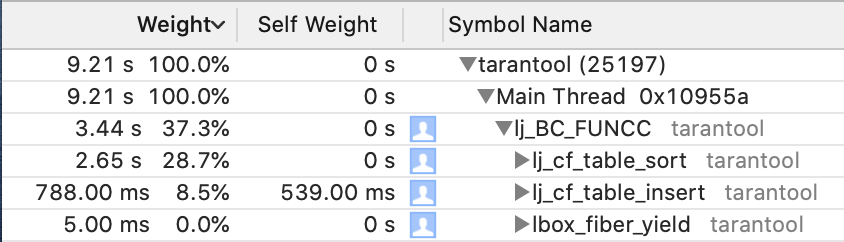The code underperforms on inserting observations into the corresponding array (table.insert call) and on buffer sorting (table.sort). That’s where ffi (foreign function interface) comes to the rescue. Ffi allows accessing functions from the C standard library and working with them in Lua as if they were routine Lua objects (well, almost; for example, while table indexing in Lua starts with 1, arrays created with C would still start with 0).

2. The Lua + ffi version involved building an array of double values instead of creating a buffer:

## Go

 local ffi = require('ffi')…array = ffi.new('double[?]', max_samples)for i = 0, max_samples - 1 do    array[i] = math.hugeend

We will sort this array using the C standard library:

## Go

 ffi.cdef[[

## Go

 void qsort(void *base, size_t nitems, size_t size, int (*compare)(const void *, const void*));int cmpfunc (const void * a, const void * b);

Let’s write a comparator function for double values in C and include it as a dynamic library. Here is the comparator function:

## Go

 int cmpfunc (const void * a, const void * b) {    if (*(double*)a > *(double*)b)        return 1;    else if (*(double*)a < *(double*)b)        return -1;    else        return 0;}

Now let’s build it:

## Go

 gcc -c -o metrics/quantile.o metrics/quantile.cgcc -shared -o metrics/libquantile.so metrics/quantile.o

Then we’ll include the library in our Lua code:

## Go

 local dlib_path = package.search('libquantile', package.cpath)local dlib = ffi.load(dlib_path)

Now we can populate the double array and invoke its sorting:

## Go

 local DOUBLE_SIZE = ffi.sizeof('double')ffi.C.qsort(array, len, DOUBLE_SIZE, dlib.cmpfunc)

Tests showed a 3x increase in performance, with insertion time averaging up to 30 seconds. This time, the code underperformed because Lua tables do not have a fixed size, and element types are not predefined, either. Although this allows for more flexibility in table processing, it notably reduces performance. With ffi, you can switch from Lua tables to fixed-size C arrays, so that inserting and calculating array size costs O(1) instead of O(log n). Sorting is also much faster due to the fixed types and, therefore, fixed element sizes. But this solution introduces a GCC dependency that complicates application delivery. So we had to get rid of the C code.

3. Lua + ffi + homebrew sorting. The simplest quicksort in Lua turned out to run only a couple of seconds longer than our previous version involving a C library. This result was good enough for us, especially since it didn’t depend on GCC, so we decided to stop here.

The last step was to add quantile rotation using the sliding window algorithm. We create a ring queue consisting of several collectors (5, for example) and make one of them the leading one (head). Monitored values are written to each of these collectors. After the specified time has expired (60 seconds, for instance), the head collector is reset and the next one in the queue becomes the new head. The quantile value is fetched from the current head only. This approach ensures that the data are kept up-to-date because, without a sliding window, the values would be calculated over the entire period.

### Memory Usage

metrics.quantile uses two arrays:

• A buffer of max_samples * sizeof(double) = 500 × 8 bytes.
• An observation array of (2 * max_samples + 2) * sizeof(struct sample) = 1002 × 16 bytes. The size of the observation array can increase when the observed values vary by several orders of magnitude.

There are age_buckets_count collectors created in metrics.summary, so the total size is:

age_buckets_count * (max_samples * sizeof(double) + (2 * max_samples + 2) * sizeof(struct sample)) = 5 × (500 × 8 + 1002 × 16) bytes, or about 100 KB.

### Performance Impact

We performed load testing with Yandex.Tank. With all application metrics turned off, the results read as follows: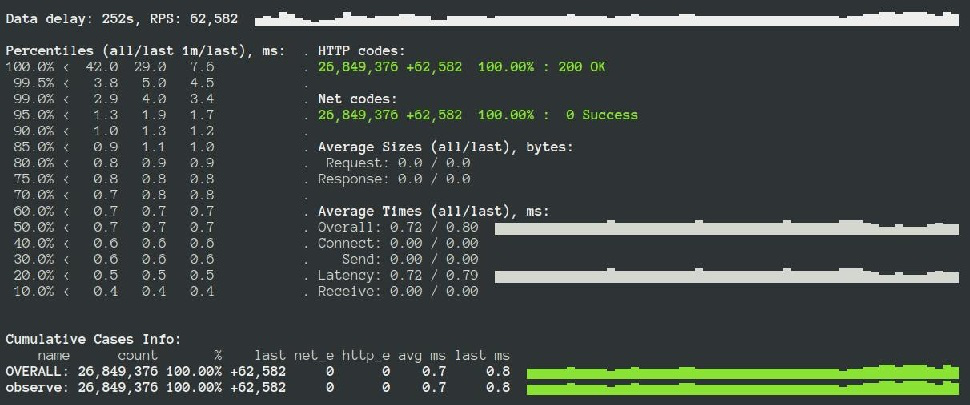With our summary collector: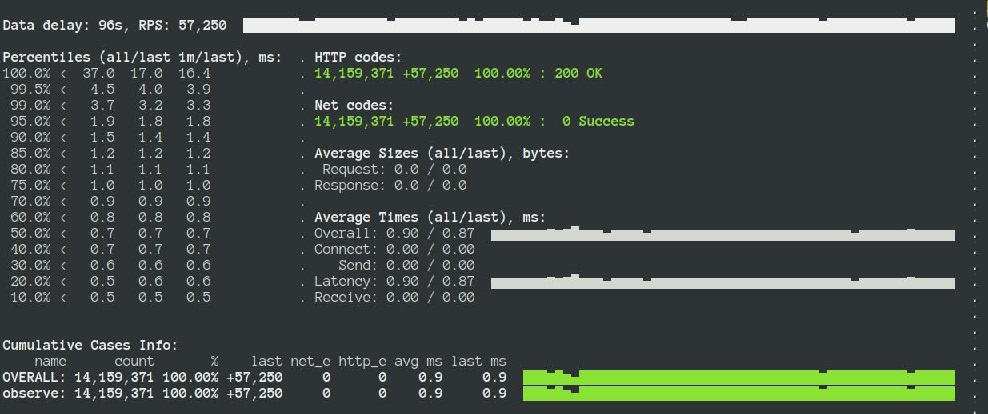Performance dropped by ~10%, which is a cost you have to pay for using metrics. If you want to avoid significant drawdown, you might want to use the collector carefully, for instance, measure only a portion of requests.

## Go

 tarantoolctl rocks install metrics 0.10.0

## Go

 local metrics = require('metrics') -- attaching metrics -- Creating a summary collectorlocal http_requests_latency = metrics.summary(    'http_requests_latency', 'HTTP requests latency',    {[0.5]=0.01, [0.9]=0.01, [0.99]=0.01},    {max_age_time = 60, age_buckets_count = 5}) -- Monitoring a valuelocal latency = math.random(1, 10)http_requests_latency:observe(latency)

Export to JSON, Prometheus, and Graphite is supported. Here is what the collected results might look like in Grafana: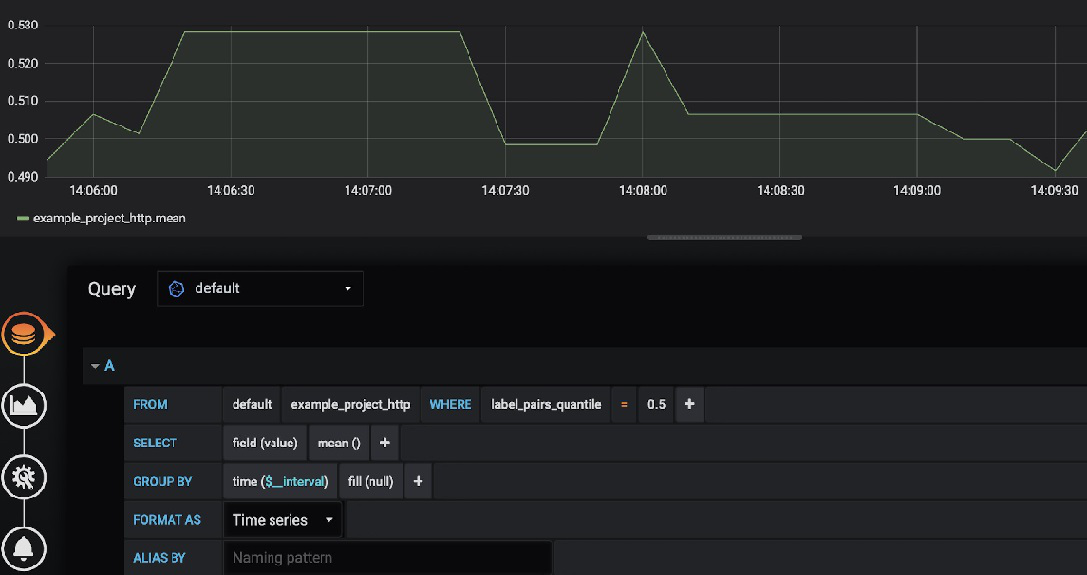### Conclusion

We wrote a summary collector for tarantool/metrics. During development, we encountered a performance challenge, which we solved using ffi. You can use the new collector to monitor values that may benefit from keeping track of quantiles, such as HTTP request latency. The summary collector can be applied in any Tarantool-based product where service response time is critical, like data-intensive applications where large amounts of data are accessed via HTTP requests. Monitoring this metric will help you understand what requests are straining your system.